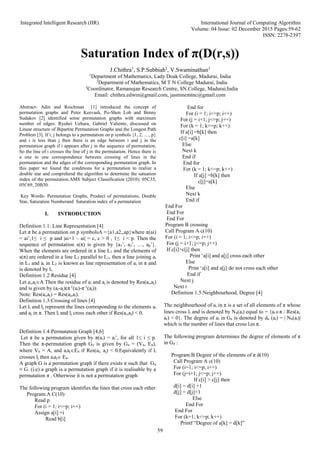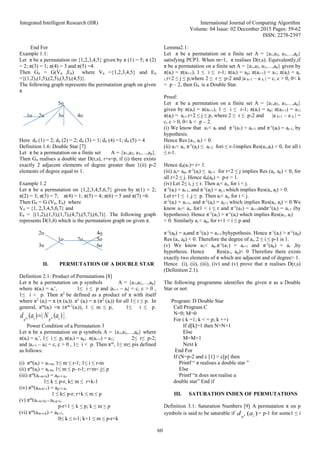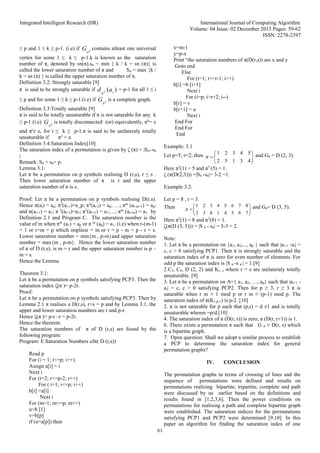Successfully reported this slideshow.

# Saturation Index of π(D(r,s))×

# Saturation Index of π(D(r,s))

Abstract-Adin and Roichman  introduced the concept of permutation graphs and Peter Keevash, Po-Shen Loh and Benny Sudakov  identified some permutation graphs with maximum number of edges. Ryuhei Uehara, Gabriel Valiente, discussed on Linear structure of Bipartite Permutation Graphs and the Longest Path Problem . If i, j belongs to a permutation on p symbols {1, 2, …, p} and i is less than j then there is an edge between i and j in the permutation graph if i appears after j in the sequence of permutation. So the line of i crosses the line of j in the permutation. Hence there is a one to one correspondence between crossing of lines in the permutation and the edges of the corresponding permutation graph. In this paper we found the conditions for a permutation to realize a double star and comprehend the algorithm to determine the satuation index of the permutation.AMS Subject Classification (2010): 05C35, 05C69, 20B30.

Abstract-Adin and Roichman  introduced the concept of permutation graphs and Peter Keevash, Po-Shen Loh and Benny Sudakov  identified some permutation graphs with maximum number of edges. Ryuhei Uehara, Gabriel Valiente, discussed on Linear structure of Bipartite Permutation Graphs and the Longest Path Problem . If i, j belongs to a permutation on p symbols {1, 2, …, p} and i is less than j then there is an edge between i and j in the permutation graph if i appears after j in the sequence of permutation. So the line of i crosses the line of j in the permutation. Hence there is a one to one correspondence between crossing of lines in the permutation and the edges of the corresponding permutation graph. In this paper we found the conditions for a permutation to realize a double star and comprehend the algorithm to determine the satuation index of the permutation.AMS Subject Classification (2010): 05C35, 05C69, 20B30.